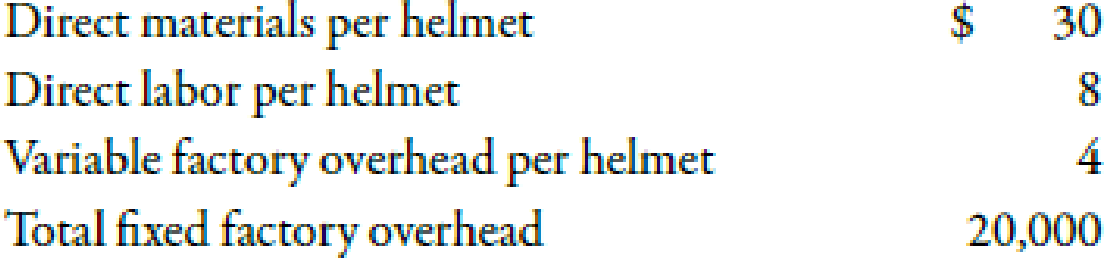Chapter 7, Problem 13BEA### Managerial Accounting: The Corners...

7th Edition
Maryanne M. Mowen + 2 others
ISBN: 9781337115773

#### Solutions

Chapter
Section### Managerial Accounting: The Corners...

7th Edition
Maryanne M. Mowen + 2 others
ISBN: 9781337115773
Textbook Problem
1 views

# Variable Cost, Fixed Cost, Contribution Margin Income StatementHead-First Company plans to sell 5,000 bicycle helmets at $75 each in the coming year. Product costs include:Variable selling expense is a commission of$3 per helmet; fixed selling and administrative expense totals \$29,500.Required: 1. Calculate the total variable cost per unit. 2. Calculate the total fixed expense for the year. 3. Prepare a contribution margin income statement for Head-First Company for the coming year.

1.

To determine

Compute per unit variable cost.

Explanation

Variable Cost:

Variable cost is the cost which changes with the change in the level of output. In other words, variable cost increases with the increase in units produced and decreases with the decrease in units produced.

Use the following formula to compute the total variable cost per unit:

Variable Cost Per Unit=Direct Material+Direct Labor+Variable Factory Overhaed+Va

2.

To determine

Compute the total fixed expense for the year.

3.

To determine

Make contribution margin income statement for Company H.

### Still sussing out bartleby?

Check out a sample textbook solution.

See a sample solution

#### The Solution to Your Study Problems

Bartleby provides explanations to thousands of textbook problems written by our experts, many with advanced degrees!

Get Started

#### Find more solutions based on key concepts